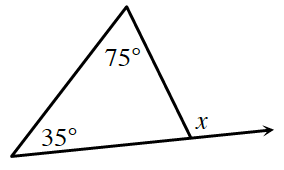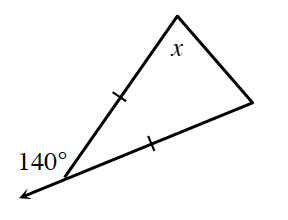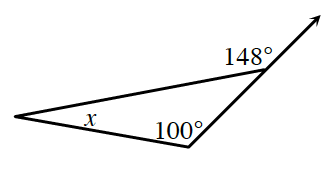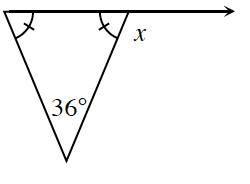### Home > INT2 > Chapter 2 > Lesson 2.1.3 > Problem2-28

2-28.

Solve for $x$ in each diagram below.

The Triangle Angle Sum Theorem states that the sum of all the interior angles in a triangle will be $180^\circ$.
Find the missing angle in each triangle.

The sum of supplementary angles is $180^\circ$.
Solve for $x$ using the general equation $\text{missing angle}+x=180^{\circ}$.

1.$x = 110^\circ$

1.$x = 70^\circ$

1.1.